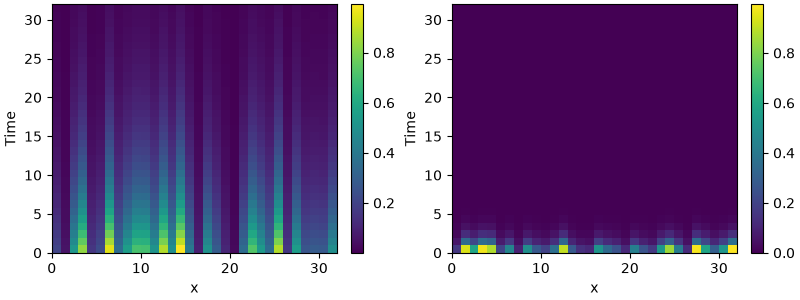# 2.15. Writing and reading trajectory data

This example illustrates how to store intermediate data to a file for later post-processing. The storage frequency is an argument to the tracker.```from tempfile import NamedTemporaryFile

import pde

# define grid, state and pde
grid = pde.UnitGrid()
state = pde.FieldCollection(
[pde.ScalarField.random_uniform(grid), pde.VectorField.random_uniform(grid)]
)
eq = pde.PDE({"s": "-0.1 * s", "v": "-v"})

# get a temporary file to write data to
path = NamedTemporaryFile(suffix=".hdf5")

# run a simulation and write the results
writer = pde.FileStorage(path.name, write_mode="truncate")
eq.solve(state, t_range=32, dt=0.01, tracker=writer.tracker(1))

# read the simulation back in again